Start typing, then use the up and down arrows to select an option from the list.# Natural Logarithmic Functions

Jules Bruno
136views
1
so the natural logarithms sometimes shortened to just Ln so Alan is the natural longer rhythm off a value is the exponents which e must be raised to determine that number. So, for example, Ellen of 1000 equals 6.908 e is the inverse of the natural log. So we do e to 6.908 That would give me 1000. Okay, so here, the inverse of the natural logarithms again represent, symbolized by the variable e can be seen as the opposite of the natural logarithms. And so, Alan of X equals y. So the inverse of my natural law y equals e to the y equals X. All right, so So applying this logic, the example below would go as follows. So we have Ellen of two equals 20.693 So the inverse of my Ln inverse of my natural law, which is e to the 0.693 equals two. Now, when do we have to deal with this natural logarithms? We're going to say that this is very common when we deal with chemical kinetics. So we deal with the chapter dealing with chemical kinetics. You will see Ln being used now, using this logic, we can help to solve this question. Here, here it says, based on your understanding of natural law, go rhythmic functions solve for the missing variable in the following question. So they give us Ellen of acts equals negative. 2.13 times 10 to the negative one times 12. plus Allen of 1 to 5. So we're gonna do here is we're gonna bring down Al Innovex. We're going to multiply these together. Don't forget the negative sign that's there. So we multiply those two together we get negative 26199 Then we're gonna add Allen of 1 to 5 to that. So punch in on your calculator Ln off 1. in your calculated, you might have to hit second function. Look for Ln and then parentheses. 1.25 close parentheses. When you do that, you'll get 0. And then when we add those together we get negative 2.39676 which is still equal toe Elena backs. We need to figure out what X is to do that we have to take the inverse of my natural logarithms. So here, when we take the inverse, that'll get rid of my Ln and then it's gonna be e to this value. So whatever the value is, it gets raised to a power. So when you're calculating, you may see this button e to the X. Usually you have to hit second function on your calculator, then hit that button, then parentheses. Plug in negative. 239676 Close parentheses. If you do it correctly, what you're gonna get is 2396760. That would be our value. Here, here, we're not gonna worry about Sig Figs. Let's just worry about getting the correct answer. So this will be my value for X Again. We use the inverse of the natural law algorithm if we have Ln X equals some value. To find the X, we have to get rid of the l N. And we do that by taking the inverse of both sides taking the inverse gets rid of the L N. And then it raises this number. Tow a power. It becomes e to that number that will help us find our missing variable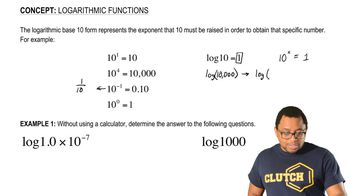03:37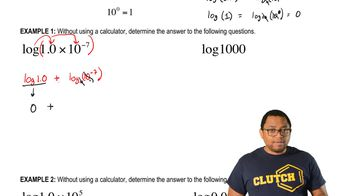01:34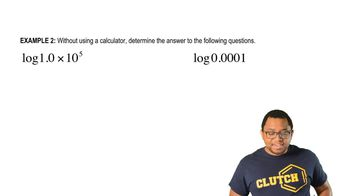02:16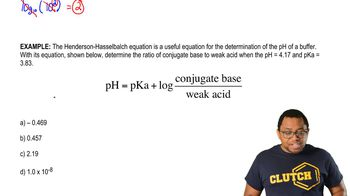04:24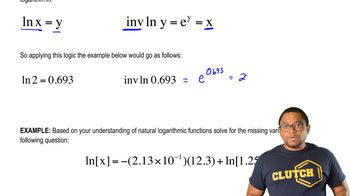03:54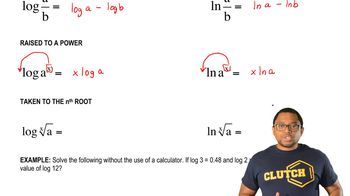04:56